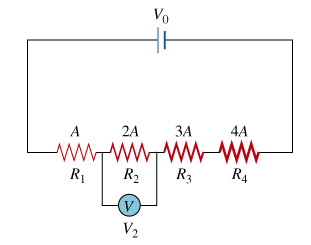# Problem: Four wires are made of the same highly resistive material, cut to the same length, and connected in series.Wire 1 has resistance R1 and cross-sectional area A.Wire 2 has resistance R2 and cross-sectional area 2A.Wire 3 has resistance R3 and cross-sectional area 3A.Wire 4 has resistance R4 and cross-sectional area 4A.A voltage V0 is applied across the series, as shown in the figure.Find the voltage V2 across wire 2.Give your answer in terms of V0, the voltage of the battery.

###### FREE Expert Solution

Resistance:

$\overline{){\mathbf{R}}{\mathbf{=}}\frac{\mathbf{\rho }\mathbf{L}}{\mathbf{A}}}$

R1 = ρL/A

R2 = ρL/2A = R1/2

R3 = ρL/3A = R1/3

R4 = ρL/4A = R1/4

88% (347 ratings)###### Problem Details

Four wires are made of the same highly resistive material, cut to the same length, and connected in series.

1. Wire 1 has resistance R1 and cross-sectional area A.
2. Wire 2 has resistance R2 and cross-sectional area 2A.
3. Wire 3 has resistance R3 and cross-sectional area 3A.
4. Wire 4 has resistance R4 and cross-sectional area 4A.

A voltage V0 is applied across the series, as shown in the figure.Find the voltage V2 across wire 2.

Give your answer in terms of V0, the voltage of the battery.

Frequently Asked Questions

What scientific concept do you need to know in order to solve this problem?

Our tutors have indicated that to solve this problem you will need to apply the Solving Resistor Circuits concept. You can view video lessons to learn Solving Resistor Circuits. Or if you need more Solving Resistor Circuits practice, you can also practice Solving Resistor Circuits practice problems.

What professor is this problem relevant for?

Based on our data, we think this problem is relevant for Professor Soldatenko's class at Los Angeles Pierce College.